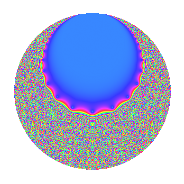# Properties

 Label 5.18.bLevel 5 Weight 18 Character orbit b Rep. character $$\chi_{5}(4,\cdot)$$ Character field $$\Q$$ Dimension 8 Newforms 1 Sturm bound 9 Trace bound 0

# Related objects

## Defining parameters

 Level: $$N$$ = $$5$$ Weight: $$k$$ = $$18$$ Character orbit: $$[\chi]$$ = 5.b (of order $$2$$ and degree $$1$$) Character conductor: $$\operatorname{cond}(\chi)$$ = $$5$$ Character field: $$\Q$$ Newforms: $$1$$ Sturm bound: $$9$$ Trace bound: $$0$$

## Dimensions

The following table gives the dimensions of various subspaces of $$M_{18}(5, [\chi])$$.

Total New Old
Modular forms 10 10 0
Cusp forms 8 8 0
Eisenstein series 2 2 0

## Trace form

 $$8q - 579096q^{4} + 379200q^{5} + 357816q^{6} - 234916344q^{9} + O(q^{10})$$ $$8q - 579096q^{4} + 379200q^{5} + 357816q^{6} - 234916344q^{9} + 329570200q^{10} + 463296576q^{11} - 29937907992q^{14} + 30646226400q^{15} + 30848001568q^{16} - 20615713280q^{19} - 47558579400q^{20} - 75039699024q^{21} + 1768741136160q^{24} - 1789249435000q^{25} - 838901194224q^{26} - 4079017824720q^{29} + 2416984007400q^{30} + 11329328658496q^{31} - 36406243632832q^{34} + 4019663899200q^{35} + 59729752432728q^{36} + 40318460422272q^{39} - 209747532172000q^{40} + 97217252847456q^{41} - 116357853210912q^{44} - 366841998003600q^{45} + 1081224261700136q^{46} - 856574357621656q^{49} - 1283266301910000q^{50} + 2468309514424896q^{51} - 3408409774777680q^{54} - 2042713226757600q^{55} + 8363016326678880q^{56} - 1091409512240640q^{59} - 11479379108104800q^{60} + 8064731010774976q^{61} - 3616160324265856q^{64} - 11989509557901600q^{65} + 27318846906958752q^{66} - 12078989597365008q^{69} - 13190931213697800q^{70} + 25241492058140736q^{71} - 29902523510328912q^{74} + 3839235716880000q^{75} + 20767634734678560q^{76} + 3229852337730880q^{79} + 1263407265391200q^{80} - 49353541005202632q^{81} + 101439947332382688q^{84} + 25693702369787200q^{85} - 165112838769552744q^{86} + 92963987535626640q^{89} + 206315814421823400q^{90} - 225591670236809664q^{91} + 162612564681867848q^{94} + 204319715505252000q^{95} - 654303222993538944q^{96} - 56327331239952768q^{99} + O(q^{100})$$

## Decomposition of $$S_{18}^{\mathrm{new}}(5, [\chi])$$ into irreducible Hecke orbits

Label Dim. $$A$$ Field CM Traces $q$-expansion
$$a_2$$ $$a_3$$ $$a_5$$ $$a_7$$
5.18.b.a $$8$$ $$9.161$$ $$\mathbb{Q}[x]/(x^{8} + \cdots)$$ None $$0$$ $$0$$ $$379200$$ $$0$$ $$q+\beta _{1}q^{2}-\beta _{2}q^{3}+(-72387+\beta _{3}+\cdots)q^{4}+\cdots$$